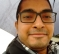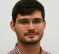## Noise Generation ConfusionStarted by 6 years ago5 replieslatest reply 6 years ago127 views

Hi,

I have to generate AWGN noise whose power is -100 dBm

I do the following calculation in MATLAB.

np_dBm = -100; % desired noise power in dBm
np_watts = 10^(-13); % noise power in watts
L = 1000; % length of noise signal
n = np_watts*(randn(1,L)+1i*randn(1,L));% computed noise
computed_noise_powerindBm = 10*log10((sum(abs(n).^2)/(length(n)))/(1/1000));

But my computed_noise_powerindBm never comes even near -100 dBm !

Sampling rate is 20 MHz, but I am not sure how will it help here.

What fundamental mistake I am doing !!

Regards

Sumit

[ - ]The error is in the computation of n:

n = sqrt(np_watts/2)* ...

because here you compute an amplitude, and the power of the complex number (Expectation) is 2.

[ - ]Okk thats solved the issue.. thanks much :)

[ - ]Hi Sumit,

1) Why are you generating a complex noise? Is there any particular reason? You can generate a real-valued Gaussian noise simply by:

noise = randn(1,L);

2) Now you need to normalize it. Do you want your power to be peak power or rms power? By the calculations you provide I suppose you want rms. Normalize your noise by its own rms like this:

noise = noise/rms(noise);

3) Now you want to calibrate it to -100 dB:

noise = noise * 10^( -100/20 );

4) Now check your rms value in dB:

rms_dB = 20*log10(rms(noise));

5) Is it just a programming task or are you gonna use a real DAC to output the noise to some physical device? Remember that above calculations work only if you assume that a signal with rms of 0 dB corresponds to a physical signal power of 0 dBm. If your physical system is calibrated to a different value, you need to subtract it. Below you have an example of the full code that would work in a more general case:

calibrationLevel_dBm = 23.5; % physical dBm power output when the digital signal has rms of 0 dB

desiredNoisePower_dBm = -100; % desired power of the noise at the output in dBm

noiseLength = 1000; % length of the noise in samples

noise = randn(1,noiseLength);

noise = noise/rms(noise) * 10^( (desiredNoisePower_dBm - calibrationLevel_dBm)/20 );

rms_dBFS = 20*log10(rms(noise)); % rms in dB Full Scale (re. digital 1) of the digital signal;

rms_dBm = rms_dBFS + calibrationLevel_dBm; % digital rms adjusted for the calibration correctionn to account for the output of the physical device

I am an audio person so I usually operate on dB SPL, but the math is exactly the same. However, if I made any error, please correct me.

Cheers,

Borys

[ - ]I work with complex signals (wireless comm). Hence I need complex noise. Your detailed information was very much helpful. Thanks a lot for your time ! :)

Regards

Sumit

[ - ]